Successfully reported this slideshow.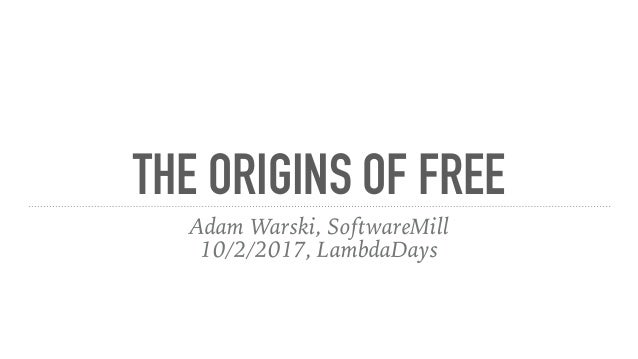Upcoming SlideShare
×

of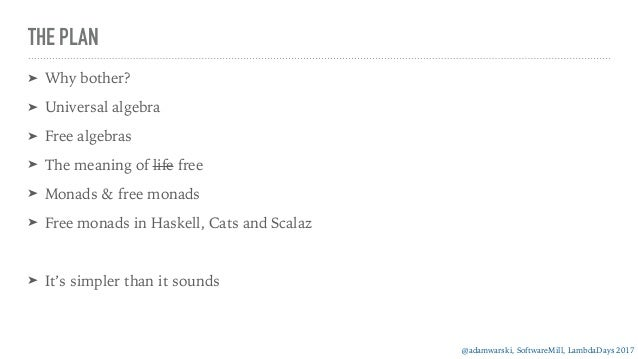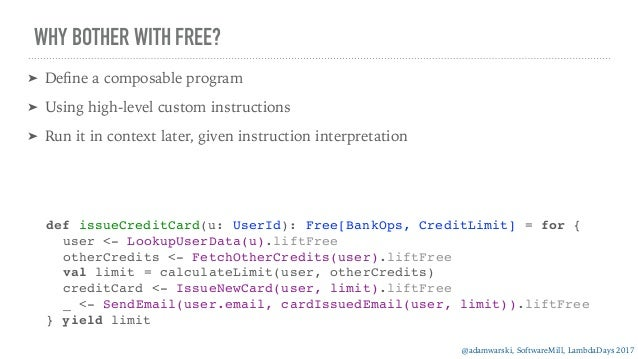Upcoming SlideShare
Jednorożce to kobiety a nie firmy. O √kobiecym w STEM
Next

3 Likes

Share

# Origins of free

What does "free", as in "free monad" mean? How can a free structure be constructed? How does this construction relate to the definition of free found in Scalaz/Cats/Haskell?

See all

See all

### Origins of free

2. 2. @adamwarski, SoftwareMill, LambdaDays 2017 THE PLAN ➤ Why bother? ➤ Universal algebra ➤ Free algebras ➤ The meaning of life free ➤ Monads & free monads ➤ Free monads in Haskell, Cats and Scalaz ➤ It’s simpler than it sounds
3. 3. @adamwarski, SoftwareMill, LambdaDays 2017 WHY BOTHER WITH FREE? ➤ Deﬁne a composable program ➤ Using high-level custom instructions ➤ Run it in context later, given instruction interpretation def issueCreditCard(u: UserId): Free[BankOps, CreditLimit] = for {  user <- LookupUserData(u).liftFree  otherCredits <- FetchOtherCredits(user).liftFree  val limit = calculateLimit(user, otherCredits)  creditCard <- IssueNewCard(user, limit).liftFree   _ <- SendEmail(user.email, cardIssuedEmail(user, limit)).liftFree  } yield limit
4. 4. @adamwarski, SoftwareMill, LambdaDays 2017 WHY BOTHER WITH FREE? def issueCreditCard(u: UserId): Free[BankOps, CreditLimit] = for {  user <- LookupUserData(u).liftFree  otherCredits <- FetchOtherCredits(user).liftFree  val limit = calculateLimit(user, otherCredits)  creditCard <- IssueNewCard(user, limit).liftFree   _ <- SendEmail(user.email, cardIssuedEmail(user, limit)).liftFree  } yield limit val productionInterpreter: BankOp ~> Future = {  override def apply[A](bo: BankOp[A]): Future[A] = bo match {  case LookupUserData(u) => oracleDB2dao.lookupUser(u)  case FetchOtherCredits(user) =>   legacySoapSystem.fetchCredits(user)  // …  }  }
5. 5. @adamwarski, SoftwareMill, LambdaDays 2017 WHY BOTHER WITH FREE? val testInterpreter: BankOp ~> Id = {  override def apply[A](bo: BankOp[A]): Id[A] = bo match {  case LookupUserData(u) => new User(…)  case FetchOtherCredits(user) => List(Credit(1000000.usd))  // …  }  } def issueCreditCard(u: UserId): Free[BankOps, CreditLimit] = for {  user <- LookupUserData(u).liftFree  otherCredits <- FetchOtherCredits(user).liftFree  val limit = calculateLimit(user, otherCredits)  creditCard <- IssueNewCard(user, limit).liftFree   _ <- SendEmail(user.email, cardIssuedEmail(user, limit)).liftFree  } yield limit
6. 6. @adamwarski, SoftwareMill, LambdaDays 2017 WHY BOTHER WITH FREE? val result: CreditLimit = issueCreditCard(UserId(42))  .foldMap(testInterpreter) def issueCreditCard(u: UserId): Free[BankOps, CreditLimit] = for {  user <- LookupUserData(u).liftFree  otherCredits <- FetchOtherCredits(user).liftFree  val limit = calculateLimit(user, otherCredits)  creditCard <- IssueNewCard(user, limit).liftFree   _ <- SendEmail(user.email, cardIssuedEmail(user, limit)).liftFree  } yield limit
7. 7. @adamwarski, SoftwareMill, LambdaDays 2017 ABOUT ME ➤ Software Engineer, co-founder @ ➤ Mainly Scala ➤ Open-source: Quicklens, MacWire, ElasticMQ, ScalaClippy, … ➤ Long time ago: student of Category Theory
8. 8. @adamwarski, SoftwareMill, LambdaDays 2017 WHAT IS AN ALGEBRA? “algebra is the study of mathematical symbols and the rules for manipulating these symbols"  Wikipedia, 2017 “the part of mathematics in which letters and other general symbols are used to represent numbers and quantities in formulae and equations."  Google Search, 2017 y = ax + b E = mc2 f(10◊x) = K(▸9) def sum(l: List[L]) = l.fold(_ + _) main = getCurrentTime >>= print
9. 9. @adamwarski, SoftwareMill, LambdaDays 2017 UNIVERSAL ALGEBRA: SIGNATURE ➤ Goal: Model programs as algebras ➤ Let’s generalise! ➤ Studies algebraic structures, rather than concrete models algebraic signature ⌃ = (S, ⌦) set S family ⌦ of sets indexed by S⇤ ⇥ S ➤ Syntax: ➤ type names: ➤ operation names:
10. 10. @adamwarski, SoftwareMill, LambdaDays 2017 UNIVERSAL ALGEBRA: SIGNATURE EXAMPLE toString(succ(0) + succ(succ(0))) S = {int, str} ⌦✏,int = {0} ⌦int,int = {succ} ⌦(int,int),int = {+} ⌦(str,str),str = {++} ⌦int,str = {toString}
11. 11. @adamwarski, SoftwareMill, LambdaDays 2017 UNIVERSAL ALGEBRA: ALGEBRA ➤ A speciﬁc interpretation of the signature ➤ for each type, a set ➤ for each operation, a function between appropriate sets ⌃ = (S, ⌦), S = {int, str} and ⌦ = {0, succ, +, ++, toString} We can deﬁne a ⌃-algebra A: |A|int = {0, 1, 2, ...} = N |A|str = {”a”, ”aa”, ..., ”b”, ”ab”, ...} succA = x.x + 1 +A = xy.x + y . . .
12. 12. @adamwarski, SoftwareMill, LambdaDays 2017 TERM ALGEBRA ➤ Can we build an algebra out of pure syntax? ➤ Expressions (terms) that can be built from the signature ➤ Rather boring, no interpretation at all |T⌃|int = {0, succ(0), succ(succ(0)), ..., 0 + 0, 0 + succ(0), ...} |T⌃|str = {toString(0), toString(succ(0)), ..., toString(0)++toString(0), ...} succT⌃ (t) = succ(t), e.g. succT⌃ (succ(0)) = succ(succ(0)) +T⌃ (t1, t2) = t1 + t2 ... ⌃ = (S, ⌦), S = {int, str} and ⌦ = {0, succ, +, ++, toString} We deﬁne the term algebra T⌃:
13. 13. @adamwarski, SoftwareMill, LambdaDays 2017 TERM ALGEBRA ➤ Deﬁned inductively ➤ base: all constants are terms ➤ step: any functions we can apply on previous terms ⌃ = (S, ⌦), S = {int, str} and ⌦ = {0, succ, +, ++, toString} {0} {0, 0 + 0, succ(0), toString(0)} {0, 0 + 0, succ(0), 0 + succ(0), succ(0) + 0, succ(0) + succ(0), toString(0), toString(succ(0)), toString(0) + +toString(0)}
14. 14. @adamwarski, SoftwareMill, LambdaDays 2017 HOMOMORPHISM ➤ Homomorphism is a function between algebras ➤ For each type, functions between type interpretations ➤ Such that operations are preserved ⌃ = (S, ⌦), S = {int, str} and ⌦ = {0, succ, +, ++, toString} When A and B are ⌃-algebras, f : A ! B is a homomorphism when: fint : |A|int ! |B|int fstr : |A|str ! |B|str 8x2|A|int fint(succA(x)) = succB(fint(x)) 8xy2|A|int f(x +A y) = f(x) +B f(y) 8x2|A|int fstr(toStringA(x)) = toStringB(fint(x))
15. 15. @adamwarski, SoftwareMill, LambdaDays 2017 INITIAL ALGEBRA ⌃-algebra I is initial when for any other ⌃-algebra A there is exactly one homomorphism between them. Theorem 1 T⌃ is initial
16. 16. @adamwarski, SoftwareMill, LambdaDays 2017 INITIAL ALGEBRA Theorem 1 T⌃ is initial ⌃ = (S, ⌦), S = {int, str} and ⌦ = {0, succ, +, ++, toString} |A|int = {0, 1, 2, ...} = N |A|str = {”a”, ”aa”, ..., ”b”, ”ab”, ...} succA = x.x + 1 +A = xy.x + y . . . We can deﬁne a ⌃-algebra A: f : T⌃ ! A f(0T⌃ ) = 0A f(succT⌃ (t)) = succA(f(t)) ...
17. 17. @adamwarski, SoftwareMill, LambdaDays 2017 INITIAL ALGEBRA ➤ Only one way to interpret a term ➤ no junk: term algebra contains only what’s absolutely necessary ➤ no confusion: no two values are combined if they don’t need to be ➤ There’s only one initial algebra (up to isomorphism) ⌃-algebra I is initial when for any other ⌃-algebra A there is exactly one homomorphism between them. Theorem 1 T⌃ is initial
18. 18. @adamwarski, SoftwareMill, LambdaDays 2017 INITIAL ALGEBRA ➤ This algebra is deﬁnitely not initial: ⌃ = (S, ⌦), S = {int, str} and ⌦ = {0, succ, +, ++, toString} ➤ Junk: strings “a”, “b”, … ➤ Confusion: 0+succ(0) is same as succ(0)+0 We can deﬁne a ⌃-algebra A: |A|int = {0, 1, 2, ...} = N |A|str = {”a”, ”aa”, ..., ”b”, ”ab”, ...}
19. 19. @adamwarski, SoftwareMill, LambdaDays 2017 FREE ALGEBRA For any set X, T⌃(X) is the term algebra with X added as ”constants” (but called variables) ⌃ = (S, ⌦), S = {int, str} and ⌦ = {0, succ, +, ++, toString} Xint = {i, j, k} Xstr = {s1, s2} succ(i) + j + succ(succ(k)) s1 + +toString(0) toString(succ(0) + k) + +s2
20. 20. @adamwarski, SoftwareMill, LambdaDays 2017 FREE ALGEBRA ➤ An interpretation of the variables determines an interpretation of any term Theorem 1 For any variable set X, T⌃(X) is free For any set X, T⌃(X) is the term algebra with X added as ”constants” (but called variables) ⌃-algebra I is free over X (X ⇢ I) when for any other ⌃-algebra A, any function f : X ! |A| extends uniquely to a homomorphism f# : I ! A between them.
21. 21. @adamwarski, SoftwareMill, LambdaDays 2017 FREE ALGEBRA EXAMPLE ⌃ = (S, ⌦), S = {int, str} and ⌦ = {0, succ, +, ++, toString} Xint = {i, j, k} Xstr = {s1, s2} |A|int = N, |A|str = {”a”, ”aa”, ..., ”b”, ”ab”, ...} succA = x.x + 1 +A = xy.x + y . . . f : X ! |A| f(i) = 10, f(j) = 5, f(k) = 42 f(s1) = ”lambda”, f(s2) = ”days” f# : T⌃(X) ! A f# (toString(succ(j) + succ(0)) + +s1) = ”7lambda” f# (s2 + +toString(k) + +s2) = ”lambda42days”
22. 22. @adamwarski, SoftwareMill, LambdaDays 2017 MEANING OF FREE ➤ Free to interpret in any way ➤ no constraints ➤ Free of additional structure ➤ only what’s absolutely necessary ➤ No junk, no confusion
23. 23. @adamwarski, SoftwareMill, LambdaDays 2017 FREE RECAP ➤ Algebraic signature: ➤ All possible interpretations: algebras ➤ For any variable set ➤ The term algebra is free ➤ any interpretation of the variables ➤ determines an interpretation of any term ➤ A general construction ⌃ = (S, ⌦) X T⌃(X) f : X ! |A| f# : T⌃(X) ! A
24. 24. @adamwarski, SoftwareMill, LambdaDays 2017 MODELLING SEQUENTIAL PROGRAMS: MONADS ➤ A sequential program can: ➤ return a value (pure) ➤ compute what to do next basing on previous result (flatMap) ➤ People decided to call an object with such operations a Monad ➤ Hence, we’ll use Monads to represent programs as data ➤ + sanity laws
25. 25. @adamwarski, SoftwareMill, LambdaDays 2017 FREE MONAD ➤ Signature ~ pure + flatMap ➤ Variables ~ operations (our DSL) ➤ Free Monad ~ terms built out of pure, flatMap, our DSL ➤ modulo monad laws! ➤ e.g. flatMap(pure(x), f) = f(x) Interpretation of the DSL determines the interpretation of the whole program
26. 26. @adamwarski, SoftwareMill, LambdaDays 2017 FREE MONAD ➤ Our “world” (category) are Scala/Haskell/… monads (not algebras) ➤ The “world” (category) of the variables are generic Scala/Haskell/… terms (not sets) ➤ Signature: ➤ types: M[_] ➤ operations: ➤ pure[A]: A => M[A] ➤ flatMap[A, B](ma: M[A], f: A => M[B]): M[B] ➤ Modulo monad laws
27. 27. @adamwarski, SoftwareMill, LambdaDays 2017 FREE IN CATS/SCALAZ trait Free[F[_], A]    object Free {  case class Pure[F[_], A](a: A) extends Free[F, A]  case class Suspend[F[_], A](a: F[A]) extends Free[F, A]  case class FlatMapped[F[_], B, C](  c: Free[F, C], f: C => Free[F, B] extends Free[F, B]  }
28. 28. @adamwarski, SoftwareMill, LambdaDays 2017 FREE IN HASKELL data Free f r = Free (f (Free f r)) | Pure r trait Free[F[_], A]    object Free {  case class Pure[F[_], A](a: A) extends Free[F, A]  case class Join[F[_], A](f: F[Free[F, A]]) extends Free[S, A]  } f/F[_] must be a functor!
29. 29. @adamwarski, SoftwareMill, LambdaDays 2017 SUMMING UP ➤ Direct construction of free algebras ➤ Hand-wavy construction of free monad ➤ Free ➤ free to interpret in any way ➤ free of constraints ➤ no junk, no confusion ➤ Free in Haskell is the same free as in Scala
30. 30. @adamwarski, SoftwareMill, LambdaDays 2017 FURTHER READING ➤ “Foundations of Algebraic Speciﬁcation and Formal Software Development” by Donald Sannella and Andrzej Tarlecki ➤ The Internet
31. 31. THANK YOU!
•#### IlyaGalyetov

Aug. 10, 2017
•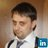#### RomanMelnyk3

Feb. 12, 2017
•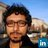#### JuanPabloRomeroMndez

Feb. 10, 2017

Total views

1,501

On Slideshare

0

From embeds

0

Number of embeds

237

25

Shares

0